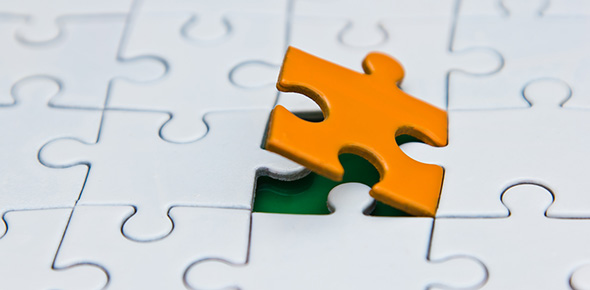# The Laws Of Thermodynamics!

18 Questions | Total Attempts: 159SettingsCreate your own Quiz.

• 1.
There are two  cups on the a table.  Blue cup- 250 ml of water at 80 degrees. Yellow cup- 250 ml of water at 60 degrees. If you moved the blue and yellow cups until they touch, in which direction will the heat be transferred?
• A.

From the yellow to the blue, they will have the same temperature after equilibrium is established.

• B.

From the blue to the yellow, they will have the same temperature after equilibrium is established.

• C.

From the yellow to the blue, the blue will be hotter than the yellow after equilibrium is established.

• D.

From the blue to the yellow, the yellow will be hotter than the yellow after equilibrium is established.

• 2.
A beaker with sulphuric acid diluted in water represents what type of system?
• A.

Closed

• B.

Open

• C.

Isolated

• D.

Option 4

• 3.
A hot water bottle filled with hot water, is an example of a ___________ system.
• A.

Closed

• B.

Open

• C.

Isolated

• D.

Option 4

• 4.
A calorimeter whose reaction vessel is full of a gaseous mixture.
• A.

Closed

• B.

Open

• C.

Isolated

• D.

Option 4

• 5.
Indicate whether the temperature in the calorimeter would increase or decrease in the following situations. In a reaction chamber, water and metallic sodium react violently, sometimes bursting into flames
• A.

Decreases

• B.

Increases

• C.

Option 3

• D.

Option 4

• 6.
Indicate whether the temperature in the calorimeter would increase or decrease. When dry ice (solid carbon dioxide) is in a reaction chamber, it solidifies at -78.5 degrees.
• A.

Increase

• B.

Decrease

• C.

Option 3

• D.

Option 4

• 7.
Energy of motion is referred to as __________ energy.
• A.

Thermal

• B.

Kinetic

• C.

Chemical

• D.

Potential

• 8.
The ______ law of thermodynamics states that energy can neither be created nor destroyed.
• A.

Oth

• B.

1st

• C.

2nd

• D.

3rd

• 9.
When muscles contract, chemical energy is converted to mechanical energy with the loss of heat. This is an example of the ______ law of thermodynamics.
• A.

0th

• B.

1st

• C.

2nd

• D.

3rd

• 10.
_______ is a measure of randomness or disorder.
• A.

Heat

• B.

Energy

• C.

Entropy

• D.

Option 4

• 11.
If two objects are in contact they...
• A.

Will eventually have the same temperature

• B.

Will be in thermal equilibrium

• C.

Will have the same average kinetic energy for their particles.

• D.

All of the above

• 12.
Which law of thermodynamics is pictured in the image?
• A.

0th

• B.

1st

• C.

2nd

• D.

3rd

• 13.
Which law of thermodynamics states that if A and B are in thermal equilibrium and B and C are in thermal equilibrium then A and C must be in thermal equilibrium.
• A.

Oth

• B.

1st

• C.

2nd

• D.

3rd

• 14.
Which law states that at absolute zero the entropy of a pure crystalline solid is zero. There is no movement of particles.
• A.

0th

• B.

1st

• C.

2nd

• D.

3rd

• 15.
A thermometer comes to thermal equilibrium with whatever it is placed in, therefore a thermometer actually registers its own heat.
• A.

True

• B.

False

• 16.
If a piece of aluminum is placed in contact with both a bathtub full of cool water and a cup of hot water, which way will heat flow?
• A.

From the bathtub to the cup

• B.

From the cup to the bathtub

• C.

Option 3

• D.

Option 4

• 17.
If oil has a much lower specific heat capacity then water...
• A.

Water requires more energy to increase the temperature

• B.

Oil takes more energy to increase the temperature

• C.

Option 3

• D.

Option 4Back to top